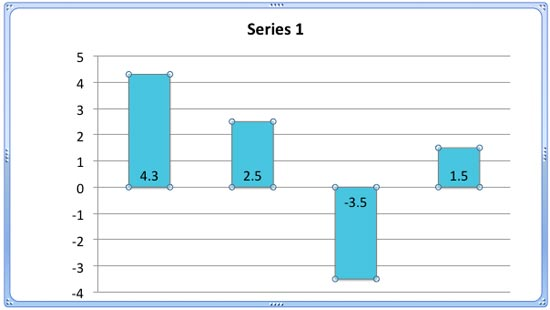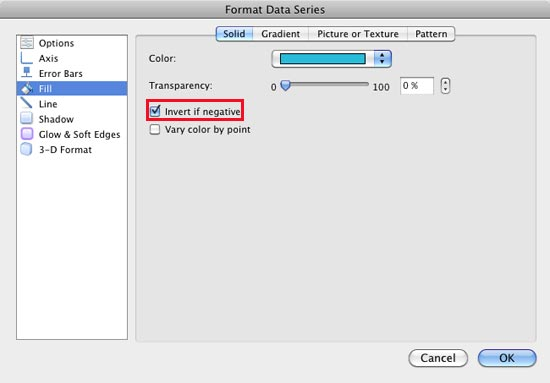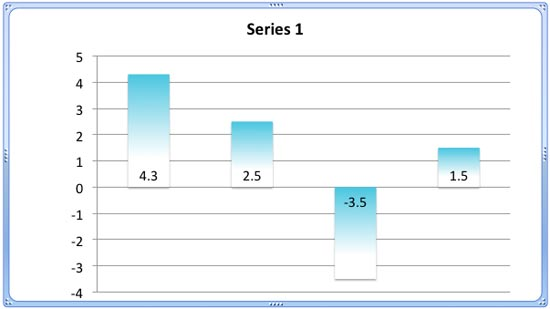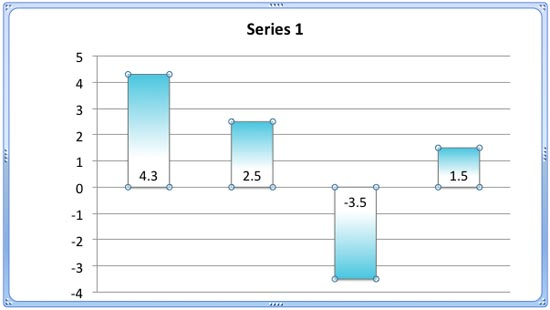# Charts in PowerPoint, Using the Invert if Negative Option in PowerPoint 2011 for Mac

Highlight negative values in a chart by using the Invert if Negative option in PowerPoint 2011 for Mac.

Author:

Product/Version: PowerPoint 2011 for Mac

OS: Mac OS X

Does your chart has any data with negative values? PowerPoint by default uses the same fill color for negative values as is used for positive values -- look at Figure 1 below where we have used solid fills for our columns representing a series. Of course, you can override this nature of PowerPoint using the Invert if Negative option, that we last explored at the end of our Changing Fills and Outlines of Charts tutorial. In this tutorial, let us explore this option further.Figure 1: Chart with negative figures

Note: The Invert if Negative option is only available for Column, Bar, Cylinder, Cone and Pyramid chart types. The Pie and Area chart types do not have this option since they don't plot negative figures.

To use the Invert if Negative option you must have inserted a chart on your slide that has negative values, as shown in Figure 1 above.

1. Select the data series including negative values by clicking on it (see Figure 2). Alternatively you can select the data series from the Chart elements drop-down list.Figure 2: Chart element (data series) with negative value selected
2. With the data series selected, select either the Chart Layout or the Format tab of the Ribbon that you can see in Figure 3. With any of these tabs selected, locate the Current Selection group that you can see in Figure 3 towards the extreme left. Within this group, make sure that the Chart Elements drop-down list (highlighted in red within Figure 3) is displaying the name of the selected Chart element. Then, click the Format Selection button below it (highlighted in blue within Figure 3).Figure 3: Format Selection button to be clicked after the proper data series is selected
3. This summons the Format Data Series dialog box. Make sure that the Fill option is selected in the sidebar as shown in Figure 4.Figure 4: Format Data Series dialog box
4. Make sure the Solid tab is selected and then select the Invert if Negative check box (highlighted in red within Figure 4). Then click the OK button. This will change the color of the negative figure column to white, as you can see in Figure 5. Compare Figures 5 and 2.Figure 5: Fill of the Negative figure column changed to white

Tip: Want to change the color of the negative figure column from white to some other color? Refer to our Changing Color for Negative Data Series of Charts in PowerPoint 2011 for Mac tutorial.
In the above example we have used the solid color as fill for the data series. If the fill is a gradient, then the result of selecting the Invert if Negative check box will be little different. Figure 6 shows a chart with gradient fill used for the data series before applying Invert if Negative.Figure 6: Chart with gradient fill applied to the columns (data series)
5. If you select the Invert if Negative check box for this chart, the result will look like something that you can see in Figure 7. Compare the third column of the chart in Figures 6 and 7.Figure 7: Negative figure column with gradient fill inverted

As you can see in Figure 7 the gradient fill of the column with negative figure has been inverted.
Note: Selecting the Invert if Negative check box won't make any difference to the negative figure columns if the picture or the texture fill has been used in them.

## Feature List 01 (Layout with Tabs)

The Feature List Layout comprises three sample PowerPoint presentations: one each to create a visual list for 4 features, 5 features, and 6 features. These work great when you have too much information to fit within one slide. This solution lets you use multiple slides, but the visual result is still that of one single slide!